Effect Size Measures for F Tests in GLM

A significant F test in a linear model indicates that the effect of the term or contrast being tested might be real. The next thing you want to know is, How big is the effect? Various measures have been devised to give answers to this question that are comparable over different experimental designs. If you specify the experimental EFFECTSIZE option in the MODEL statement, then GLM adds to each ANOVA table estimates and confidence intervals for three different measures of effect size:

• the noncentrality parameter for the F test

• the proportion of total variation accounted for (also known as the semipartial correlation ratio or the squared semipartial correlation)

• the proportion of partial variation accounted for (also known as the full partial correlation ratio or the squared full partial correlation)

The adjectives semipartial and full partial might seem strange. They refer to how other effects are partialed out of the dependent variable and the effect being tested. For semipartial statistics, all other effects are partialed out of the effect in question, but not the dependent variable. This measures the (adjusted) effect as a proportion of the total variation in the dependent variable. On the other hand, for full partial statistics, all other effects are partialed out of both the dependent variable and the effect in question. This measures the (adjusted) effect as a proportion of only the dependent variation remaining after partialing, or in other words the partial variation. Details about the computation and interpretation of these estimates and confidence intervals are discussed in the remainder of this section.

The noncentrality parameter is directly related to the true distribution of the F statistic when the effect being tested has a non-null effect. The uniformly minimum variance unbiased estimate for the noncentrality is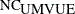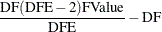where FValue is the observed value of the F statistic for the test and DF and DFE are the numerator and denominator degrees of freedom for the test, respectively. An alternative estimate that can be slightly biased but has a somewhat lower expected mean square error is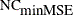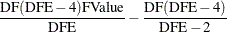(See Perlman and Rasmussen (1975), cited in Johnson, Kotz, and Balakrishnan (1994).) A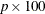% lower confidence bound for the noncentrality is given by the value of NC for which probf(FValue,DF,DFE,NC) = p, where probf() is the cumulative probability function for the non-central F distribution. This result can be used to form a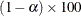% confidence interval for the noncentrality.

The partial proportion of variation accounted for by the effect being tested is easiest to define by its natural sample estimate,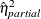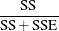where SSE is the sample error sum of squares. Note thatis actually sometimes denoted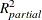or just R square, but in this context the R square notation is reserved for the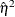corresponding to the overall model, which is just the familiar R square for the model.is actually a biased estimate of the true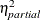; an alternative that is approximately unbiased is given by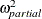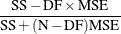where MSE = SSE/DFE is the sample mean square for error and N is the number of observations. The trueis related to the true noncentrality parameter NC by the formula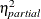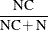This fact can be employed to transform a confidence interval for NC into one for. Note that some authors (Steiger and Fouladi; 1997; Fidler and Thompson; 2001; Smithson; 2003) have published slightly different confidence intervals for, based on a slightly different formula for the relationship betweenand NC, apparently due to Cohen (1988). Cohen’s formula appears to be approximately correct for random predictor values (Maxwell, 2000), but the one given previously is correct if the predictor values are assumed fixed, as is standard for the GLM procedure.

Finally, the proportion of total variation accounted for by the effect being tested is again easiest to define by its natural sample estimate, which is known as the (semipartial)statistic,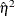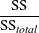where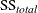is the total sample (corrected) sum of squares, and SS is the observed sum of squares due to the effect being tested. As with,is actually a biased estimate of the true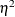; an alternative that is approximately unbiased is the (semipartial)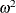statistic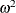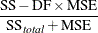where MSE = SSE/DFE is the sample mean square for error. Whereasdepends only on the noncentrality for its associated F test, the presence of the total sum of squares in the previous formulas indicates thatdepends on the noncentralities for all effects in the model. An exact confidence interval is not available, but if you write the formula foras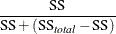then a conservative confidence interval can be constructed as for, treating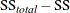as the SSE and N – DF – 1 as the DFE (Smithson, 2004). This confidence interval is conservative in the sense that it implies values of the truethat are smaller than they should be.

Estimates and confidence intervals for effect sizes require some care in interpretation. For example, while the true proportions of total and partial variation accounted for are nonnegative quantities, their estimates might be less than zero. Also, confidence intervals for effect sizes are not directly related to the corresponding estimates. In particular, it is possible for the estimate to lie outside the confidence interval.

As for interpreting the actual values of effect size measures, the approximately unbiasedestimates are usually preferred for point estimates. Some authors have proposed certain ranges as indicating small, medium, and large effects (Cohen, 1988), but general benchmarks like this depend on the nature of the data and the typical signal-to-noise ratio; they should not be expected to apply across various disciplines. For example, while anvalue of 10% might be viewed as large for psychometric data, it can be a relatively small effect for industrial experimentation. Whatever the standard, confidence intervals for true effect sizes typically span more than one category, indicating that in small experiments, it can be difficult to make firm statements about the size of effects.

Example

The data for this example are similar to data analyzed in Steiger and Fouladi (1997); Fidler and Thompson (2001); Smithson (2003). Consider the following hypothetical design, testing 28 men and 28 women on seven different tasks.

data Test;
do Task = 1 to 7;
do Gender = 'M','F';
do i = 1 to 4;
input Response @@;
output;
end;
end;
end;
datalines;
7.1 2.8 3.9 3.7     6.5 6.5 6.5 6.6
7.1 5.5 4.8 2.6     3.6 5.4 5.6 4.5
7.2 4.6 4.9 4.6     3.3 5.4 2.8 1.5
5.6 6.2 5.4 6.5     5.6 2.7 3.8 2.3
2.2 5.4 5.6 8.4     1.2 2.0 4.3 4.6
9.1 4.5 7.6 4.9     4.3 7.7 6.5 7.7
4.5 3.8 5.9 6.1     1.7 2.5 4.3 2.7
;

This is a balanced two-way design with four replicates per cell. The following statements analyze this data. Since this is a balanced design, you can use the SS1 option in the MODEL statement to display only the Type I sums of squares.

proc glm data=Test;
model Response = Gender|Task / ss1;
run;

The analysis of variance results are shown in Figure 42.15.

Figure 42.15: Two-Way Analysis of Variance

The GLM Procedure

Dependent Variable: Response

Source DF Type I SS Mean Square F Value Pr > F
Gender 1 14.40285714 14.40285714 6.00 0.0185
Task 6 38.15964286 6.35994048 2.65 0.0285
Gender*Task 6 35.99964286 5.99994048 2.50 0.0369

You can see that the two main effects as well as their interaction are all significant. Suppose you want to compare the main effect of Gender with the interaction between Gender and Task. The sums of squares for the interaction are more than twice as large, but it’s not clear how experimental variability might affect this. The following statements perform the same analysis as before, but add the EFFECTSIZE option to the MODEL statement; also, with ALPHA=0.1 option displays 90% confidence intervals, ensuring that inferences based on the p-values at the 0.05 levels will agree with the lower confidence limit.

proc glm data=Test;
model Response = Gender|Task / ss1 effectsize alpha=0.1;
run;

The Type I analysis of variance results with added effect size information are shown in Figure 42.16.

Figure 42.16: Two-Way Analysis of Variance with Effect Sizes

The GLM Procedure

Dependent Variable: Response

Source DF Type I SS Mean Square F Value Pr > F Noncentrality Parameter Total Variation Accounted For Partial Variation Accounted For
Min Var Unbiased
Estimate
Low MSE Estimate 90% Confidence Limits Semipartial Eta-Square Semipartial Omega-Square Conservative
90% Confidence Limits
Partial Eta-Square Partial Omega-Square 90% Confidence Limits
Gender 1 14.40285714 14.40285714 6.00 0.0185 4.72 4.48 0.521 17.1 0.0761 0.0626 0.0019 0.2030 0.1250 0.0820 0.0092 0.2342
Task 6 38.15964286 6.35994048 2.65 0.0285 9.14 8.69 0.870 27.3 0.2015 0.1239 0.0000 0.2772 0.2746 0.1502 0.0153 0.3277
Gender*Task 6 35.99964286 5.99994048 2.50 0.0369 8.29 7.87 0.463 25.9 0.1901 0.1126 0.0000 0.2639 0.2632 0.1385 0.0082 0.3160

The estimated effect sizes for Gender and the interaction all tell pretty much the same story: the effect of the interaction is appreciably greater than the effect of Gender. However, the confidence intervals suggest that this inference should be treated with some caution, since the lower confidence bound for the Gender effect is greater than the lower confidence bound for the interaction in all three cases. Follow-up testing is probably in order, using the estimated effect sizes in this preliminary study to design a large enough sample to distinguish the sizes of the effects.